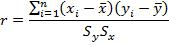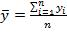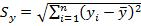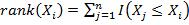If you find any of this useful, please consider donating via PayPal to help keep this site going.# More on Correlation

12/16/11

A year ago I wrote an article discussing the Pearson correlation and some issues to keep in mind when using it. I thought I'd revisit the topic of correlation, with a twist.

Recall that the sample correlation between two variables X and Y is calculated aswhereand.

With rank defined as, replace xi by rank(xi) and yi by rank(yi) in the formulas above, where I(.) is in indicator function that gives 1 if xj <= xi and 0 otherwise. What would you get? You'd actually get a very useful correlation coefficient called the Spearman correlation coefficient, which I will denote by r*.

The Spearman correlation coefficient is analogous to r and has the advantage of not being as sensitive to outlying data. It also has some disadvantages as you need to think about how to deal with ties in the ranks (and there are many ways, the midrank method being the most popular), and it is somewhat difficult to interpret in a population parameter sense. That is, r estimates the true population correlation p, but r* does not, nor does r* estimate some true population rank correlation p*, according to Gibbons and Chakraborti (2011, p. 416). Note that the SPEARMAN option in PROC CORR in SAS is not valid with the WEIGHT statement. There are many other pros and cons, but this should give a flavor of them.

In SAS the Spearman correlation coefficient can be computed by doing a PROC CORR with the SPEARMAN option like so

proc corr data=mydataset spearman;
var x y;
run;

Here is an example of a real use of it. Staff had asked me what correlation to report, r based on x and y, or r after they had transformed the data by taking the logs of x and y, or report both r's. In this case, since the log function is monotonic, the rankings would be preserved, so I mentioned that r* based on x and y or r* based on log x and log y would be identical, whereas r based on x and y and r based on log x and log y could be (and in this case was) very different. In addition, their data had a few outliers in it, so r* was a decent choice for them to consider.

The moral of the story is to really think of which type of correlation you want to use and the assumptions that are involved.